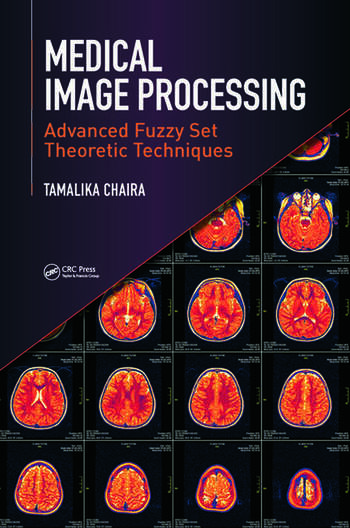# Medical Image Processing: Advanced Fuzzy Set Theoretic Techniques

## 1st Edition

Tamalika Chaira

CRC Press
Published January 28, 2015
Reference - 236 Pages - 182 B/W Illustrations
ISBN 9781498700450 - CAT# K24522

USD\$110.00

FREE Standard Shipping!

## Preview

### Summary

Medical image analysis using advanced fuzzy set theoretic techniques is an exciting and dynamic branch of image processing. Since the introduction of fuzzy set theory, there has been an explosion of interest in advanced fuzzy set theories—such as intuitionistic fuzzy and Type II fuzzy set—that represent uncertainty in a better way.

Medical Image Processing: Advanced Fuzzy Set Theoretic Techniques deals with the application of intuitionistic fuzzy and Type II fuzzy set theories for medical image analysis. Designed for graduate and doctorate students, this higher-level text:

• Provides a brief introduction to advanced fuzzy set theory, fuzzy/intuitionistic fuzzy aggregation operators, and distance/similarity measures
• Covers medical image enhancement using advanced fuzzy sets, including MATLAB®-based examples to increase contrast of the images
• Describes intuitionistic fuzzy and Type II fuzzy thresholding techniques that separate different regions/leukocyte types/abnormal lesions
• Demonstrates the clustering of unwanted lesions/regions even in the presence of noise by applying intuitionistic fuzzy clustering
• Highlights the edges of poorly illuminated images and uses intuitionistic fuzzy edge detection to find the edges of different regions
• Defines fuzzy mathematical morphology and explores its application using the Lukasiewicz operator, t-norms, and t-conorms

Medical Image Processing: Advanced Fuzzy Set Theoretic Techniques is useful not only for students, but also for teachers, engineers, scientists, and those interested in the field of medical image analysis. A basic knowledge of fuzzy set is required, along with a solid understanding of mathematics and image processing.